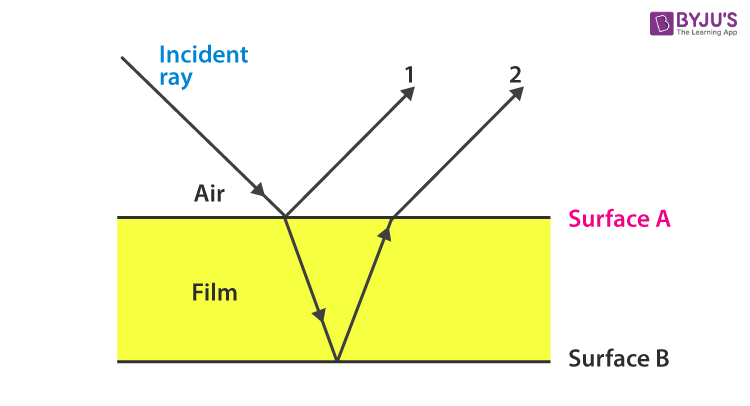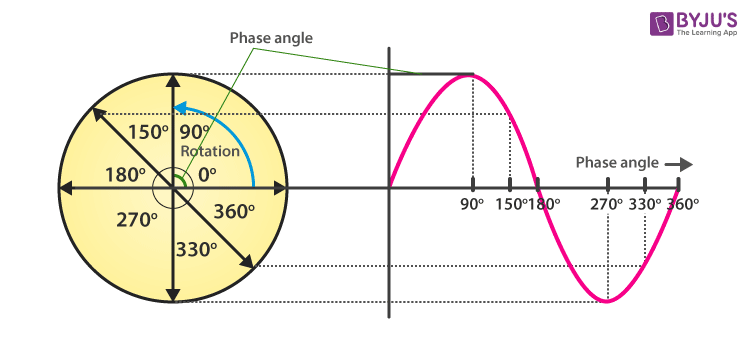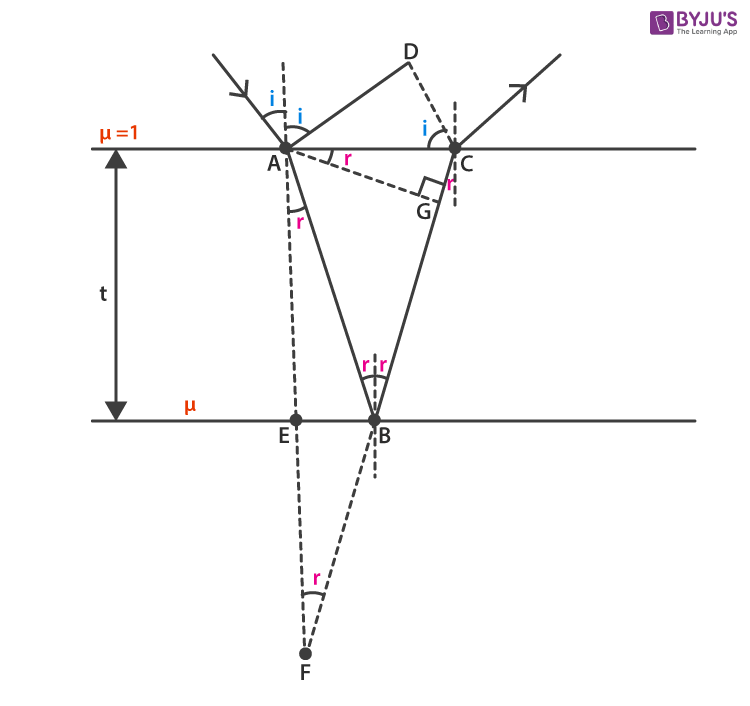Checkout JEE MAINS 2022 Question Paper Analysis : Checkout JEE MAINS 2022 Question Paper Analysis :

# Thin Film Interference

Thin-film interference is a common occurrence that happens in our everyday lives. For instance, you must have noticed some rainbow-coloured reflection in a pool of water on the side of the road or in a bubble. This observable effect is known as thin-film interference.

It occurs mostly when light is reflected several times off (interference) the sides of thin transparent material.  However, this is a simple explanation. We shall study more in-depth about this concept below.

## What is Thin Film Interference?

Thin-film interference is the phenomenon that is a result of lightwave being reflected off two surfaces that are at a distance comparable to its wavelength. When light waves that reflect off the top and bottom surfaces interfere with one another we see different coloured patterns. During this, the light reaches the boundary between two media and part of it gets reflected and some part gets transmitted.

When the second medium is a thin film, there are two reflections occurring close together at the top and bottom boundary surfaces of the thin film. Thus, there are two waves emerging from a thin film – one wave reflected off the top surface of the film and the other reflected off the bottom surface.Meanwhile, the type of interference that can take place is dependent upon these factors.

• Wavelength and angle of the incident light.
• The thickness of the film.
• Refractive indices of the material on either side of the film.
• Index of the film medium.

## Conditions for Interference

An important consideration in determining thin-film interference is also by knowing the source of the light. A source could be a monochromatic or broadband source. Monochromatic source results in interference patterns that appear as light or dark bands. Broadband source causes the interference patterns to appear as colourful bands.

We will look at the major conditions for interference and get a better idea of the concept.

The form of reflection undergo by the light waves at each boundary depends on the refraction indexes of the two mediums.

Soft reflection: Reflects off a medium with a lower refraction index, as a result, no phase shift is observed.

Hard reflection: Reflects off a medium with a higher refraction index, as a result, a phase shift is observed of λ/2.

### Reflection of Light

In this case, there is no phase change due to reflection at A and B because in both cases light is travelling from denser to rarer medium. Thus, the effective path difference between transmitted rays is also 2µt cos r.

For constructive interference,

(2n + 1) λ / 2 = 2μt Cos r

2t = mλn

For destructive interference,

nλ = 2μt Cos r

$$\begin{array}{l}2t = \left ( m +\frac{1}{2} \right )\lambda _{n}\end{array}$$

where n = 0,1,2,3..

### Transmission of Light

In this case, the phase difference between the waves is 180 degrees. Therefore,

For constructive interference,

nλ = 2μt Cos r

For destructive interference,

(2n + 1) λ / 2 = 2μt Cos r

where n = 0,1,2,3.

n is a whole number ≥0

t is the thickness of the thin film

λn is the wavelength in the medium.

## Phase Difference and Path Difference

The phase difference is the difference in phase angle between two waves. Path difference is defined as the difference in actual distance travelled by the two waves.

The phase angle is the part of one complete wave cycle measured as a fraction of 2π (360 degrees) i.e the phase difference from one wave peak to the next is 2π (360 degrees).### Relation Between Path Difference and Phase Difference

The relation between phase difference and path difference is that they are directly proportional to each other.

The phase difference Φ is 2π by wavelength (lambda) times the path difference (x)

Φ= (2π / λ) x

The interference can be constructive or destructive depending on the phase difference between the two reflected light waves, resulting in the increase or decrease in the brightness of the reflected light.

During constructive interference, the light of a particular wavelength increases in intensity whereas in destructive interference it decreases in intensity.

When light is travelling from denser medium to rarer medium, the phase shift is zero. When light is travelling from rarer medium to denser medium, the phase shift is 180 degrees.

### Derivation of Path Difference

Consider light reflecting off the top and bottom interfaces of a thin filmPath difference between the two reflected rays = µ(AB+BC) – AD

From the geometry of the triangles AEB, AFB, ABC, and ACF,

AE=EF, BC=BF &AB=BF

µ = Sin i / Sin r (Snell’s law)

In triangle ACD, Sin i = AD / AC –––––– (i)

In triangle AGC, Sin r = GC / AC –––––– (ii)

Equation (i) ÷ (ii)

Sin i / Sin r = AD / GC

Now, Path difference = µ (AB + BC) – AD = µ (AB + BC) – µGC = µ (AB + BC – GC)

Path difference = µ (BF + BC – GC) [because AB=BF]

= µ (FC – GC) = µ (FG)

Now in triangle AFG,

cos r = FG / AF

FG = AF cos r

FG = 2t cos r [because AF = AE +EF = t + t]

where t is the thickness of the thin film

Thus,

Path difference = 2μt Cos r

## Thin-film Interference Soap Bubble

When we take a soap bubble, light waves travel through the air and hits the soap film. If we look at the refractive index of air it is 1(nair = 1) whereas the film will have an index larger than 1 (nfilm > 1).

On the other hand, a 180° phase shift will be introduced in the reflected wave. It takes place at the upper boundary of the film. The shift occurs mainly because of the lower refractive index of the air than that of the film.

Further, light from the upper air-film interface will keep moving to the lower film-air interface where it is reflected. Here, the phase of the reflected wave is not changed.

## Thin-film Interference Problems and Solutions

1. A thin film of soap solution is illuminated by white light at an angle of incidence i = sin-1(4/5). In the reflected light, two dark consecutive overlapping fringes are observed corresponding to wavelengths 6.1 x 10-7 m and 6.0 x 10-7 m. The refractive index for soap solution is 4/3. Calculate the thickness of the film.

Given, µ = 4/3, λ1 = 6.1 x 10-7 m λ2 = 6.0 x 10-7 m

solution:

Sin i = 4/5

µ = Sin i / Sin r

Sin r = sin i / µ = ⅘ / 4/3

= sin r = ⅗ = 0.6

cos r = √1 – sin2r = 0.8

Given two consecutive fringes are overlapping,

1 = (n + 1)λ2

= nλ1 – nλ2 = λ2

= n = λ2 / λ1 – λ2 = 6.0 x 10-7 / 6.1 x 10-7 – 6.0 x 10-7

= 60

Now, 2μt Cos r = nλ1

t = nλ1 / 2μ Cos r = 60 x 6.1 x 10-7 / 2 x (4/3) x 0.8

t = 1.72 x 10-5 m = 1.72 x 10-2 mm

2. A soap film of refractive index 1.33 is illuminated by the light of wavelength at an angle of 45 degrees. There is complete destructive interference. For, find the thickness of the film.

Given

Refractive index = 1.33, i = 45°,

Solution:

µ = Sin i / Sin r

Sin r = sin i / µ = Sin 45 / 1.33 = (1 /√2) / 1.33

= 1/(√2)  (1.33)

Sin r = 0.5317

cos r = √1 – sin2r = 0.8496

For destructive interference

2μt Cos r = nλ

2 x 1.33 x t x 0.8469 = 1 x 5890 x 10-10 Å

t = 5890 x 10-10 / 2 x 1.33 x t x 0.8469

= 2.61410-4 mm

3. The refractive index of the thin soap film of uniform thickness is 1.4. Find the smallest thickness of the film that gives on the inteference maximum in the reflected light of wavelength 5460 amstrong fall at normal incidence.

Solution:

Maximum interference in the reflected light, in case of thin-film interference, expressed as

$$\begin{array}{l}2 \mu t cos r = \left ( 2n-1 \right )\frac{\lambda }{2}\end{array}$$

μ = Refractive index of the film relative to the surrounding

t = Thickness of film and
r = Angle of refraction

For normal incidence, r=0

$$\begin{array}{l}2\mu t = \left ( 2n-1 \right )\frac{\lambda }{2}\end{array}$$
, n=0,1,2….

For minimum thickness, n=1

$$\begin{array}{l}2\mu t_{min} = \frac{\lambda }{2}\end{array}$$

$$\begin{array}{l}t_{min} = \frac{\lambda }{4\mu }\end{array}$$

tmin = 975.

## Frequently Asked Questions on Thin Film Interference

### What affects thin-film interference?

The thin-film interference depends on the thickness of the film, light wavelength and the refractive indices.

### What is thin-film interference?

The phenomenon of thin-film interference occurs when a light wave is reflected off two surfaces at a distance equal to its wavelength. We witness varied coloured patterns as light waves reflecting off the top and bottom surfaces interfere with one another.

### What gives the colour to soap bubbles and oil spills?

The emergence of multiple colours of light from soap bubbles and oil films after reflection is due to thin-film interference.

Test Your Knowledge On Thin Film Interference!